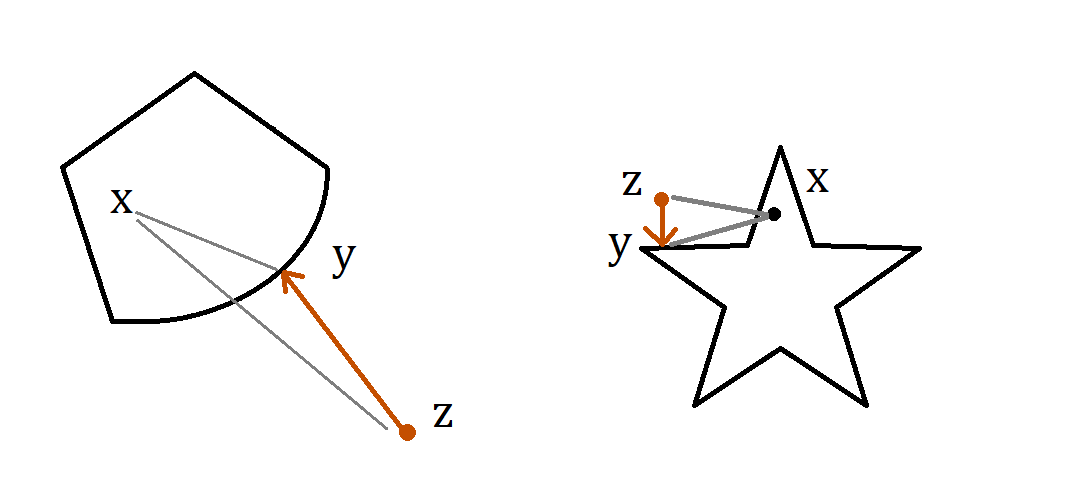# A fun convex set fact

This is actually not a new thing at all, but a thing I played with a couple years ago, and I ended up using in a numerical optimization lecture to try to impress some undergrads. They were not impressed. Since then I’ve tuned it a bit and just kind of take it for granted now, but now that I think about it, it is kind of nontrivial, and has some interesting significance. It’s come up again in the short course, so I figured I’d write it up here as well.

Statement: Suppose $\mathcal C$ is a convex set in $\mathbb R^n$, and $y = \mathbf{proj}_{\mathcal C}(z)$, for any point $z\in \mathbb R^n$. (Euclidean projection.) Then for any point $x\in \mathcal C$, $$\|y – x\|_2 \leq \|z-x\|_2.$$

Significance: This is part of a larger story that says “projecting on convex sets cannot hurt convex optimization”.  Basically, you should think of $x = x^*$ as an optimal part of some constrained optimization problem. This basically says that my contraction is a give-in, that every time I do descent-step-plus-projection, the projection step will always drive me closer to $x^*\in \mathcal C$. What I found interesting and nontrivial, though, is that this proof does not depend on any objective function $f$, so that while we really want to use this statement for $x = x^*$, it actually applies for any $x\in \mathcal C$.

Proof by picture:Note that the assumption that $\mathcal C$ is a convex set is essential; counterexamples easily exist when the set is not convex.

Proof: First, we can assume that $z\not\in \mathcal C$, since if $z\in \mathcal C$, then $z = y$ and the statement is trivially true.

Second, we should assume that $x\neq y$. Since we know that the projection on a convex set is always unique, we can encode this further and say that there exists some $\epsilon > 0$ where $\|y-z\|_2 + \epsilon \leq \|x-z\|_2$.

Now, the fact that $y$ is the projected point means that it’s the solution to the convex optimization problem
$$y = \arg\min_{y’\in \mathcal C} \; \|y’-z\|_2^2,$$ and thus we can apply the normal cone condition $-\nabla f(y)\in \mathcal N_{\mathcal C}(y)$:

$$(z-y)^T(x-y)\leq 0 \qquad\qquad \text{(normal cone condition, since x^*\in \mathcal C)}.$$

Then,

\begin{eqnarray*}
\|z-x\|_2^2 &=& \|(z-y) + (y-x)\|_2^2\\
&=& \underbrace{\|z-y\|_2^2}_{\geq 0} + \|y-x\|_2^2 + 2\overbrace{\underbrace{(z-y)^T}_{-\nabla f(z)^T}(y-x)}^{\geq 0 \text{ by N.C.}}\\
&\geq &
\|y-x\|_2^2.
\end{eqnarray*}

You may now be asking yourself, ok, but where did convexity fit in? Isn;t this only supposed to work when $\mathcal C$ is a convex set? (You can easily draw counterexamples that are nonconvex; see the star figure above.) Well, the normal cone of a nonconvex set $\mathcal S$ is the same as the normal cone of its convex hull $\mathbf{conv}(\mathcal S)$. When doing projections on $\mathcal S$ nonconvex, there is no way of ensuring that the  negative gradient will lie in the normal cone, which is a key property needed to make this proof work. But, if you are lucky and it does, then it probably means that you are taking steps that work equally well in the convex relaxation, and all will be fine! (Don’t count on it though!)

After posting findings

The extension of this statement to general Bregman divergences can be found in Nick’s course notes: https://www.cs.ubc.ca/~nickhar/F18-531/Notes20.pdf

Edit (July 2022): Thanks Mathurin Massias who  showed me how to drastically shorten the proof. (The previous version was like 5x longer.)

## One thought on “A fun convex set fact”

1.bay_baobab says:

I am impressed by the implication of that trivial looking statement. Thanks for sharing.